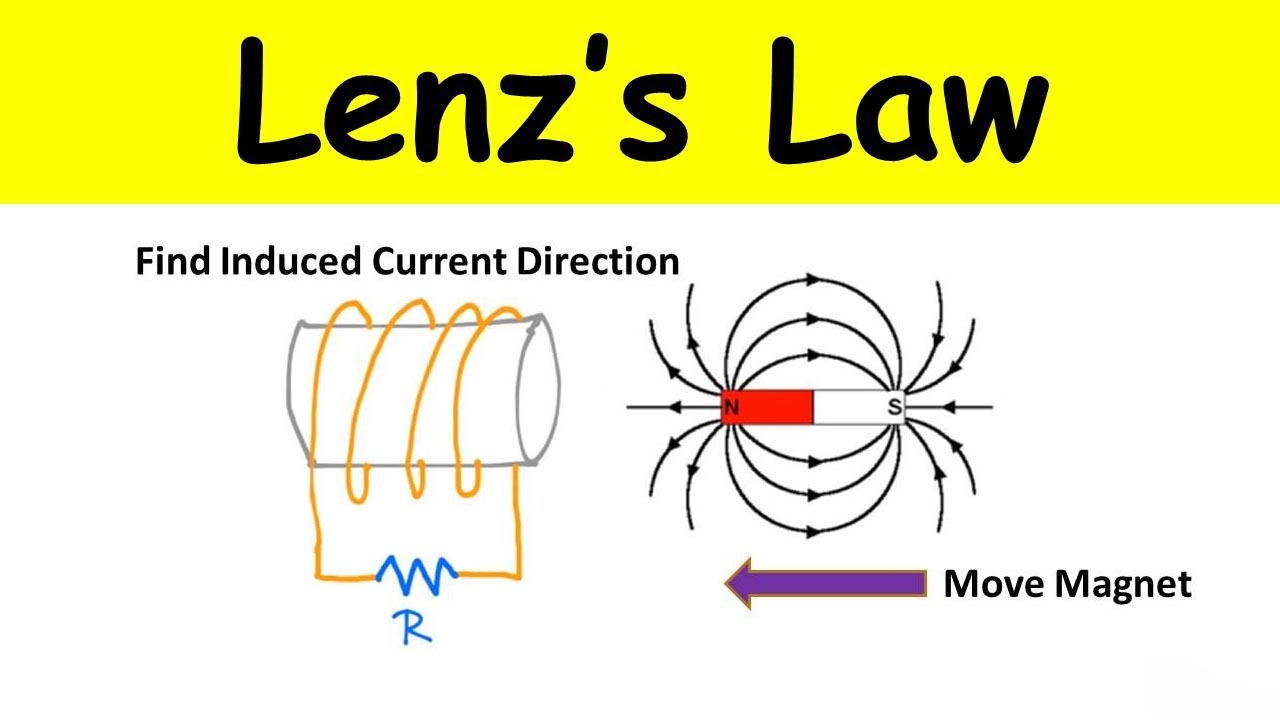## Latest Facts

### 15 Captivating Facts About Hydroponic FarmerVonni Rhea

Published: 09 Sep 2023Lenz’s Law is a fundamental principle in the field of electromagnetism that describes the behavior of induced electric fields. Named after the Russian physicist Heinrich Lenz, this law provides crucial insights into the interaction between magnetic fields and electric currents. Understanding Lenz’s Law is essential for comprehending various phenomena such as electromagnetic induction, Faraday’s Law, and the functioning of transformers, motors, and generators.

In this article, we will delve into the intriguing world of Lenz’s Law and explore ten fascinating facts that highlight its significance in the realm of physics. From the relationship between magnetic flux and induced emf to the concept of self-induction, these facts will shed light on the remarkable properties and applications of Lenz’s Law.

## Lenz’s Law is an essential principle in electromagnetism

Lenz’s Law states that the direction of an induced electric current in a conductor will always oppose the change in the magnetic field that caused it. This law is based on the principle of conservation of energy.

## Lenz’s Law is named after Heinrich Friedrich Emil Lenz

Heinrich Friedrich Emil Lenz was a Russian physicist who formulated this law in His work on electromagnetism made significant contributions to the understanding of electromagnetic fields and their effects.

## Lenz’s Law plays a crucial role in Faraday’s Law of Electromagnetic Induction

Faraday’s Law of Electromagnetic Induction states that a change in the magnetic field through a conductor induces an electromotive force (EMF) and, subsequently, an electric current. Lenz’s Law determines the direction of this induced current.

## Lenz’s Law follows the principle of Newton’s Third Law of Motion

Lenz’s Law can be understood through the application of Newton’s Third Law of Motion, which states that every action has an equal and opposite reaction. The induced current in a conductor is the reaction to changes in the magnetic field.

## Lenz’s Law is used in various applications

Lenz’s Law has practical applications in many fields such as power generation, electric motors, transformers, and magnetic levitation. Understanding this law allows engineers to optimize the design and efficiency of these devices.

## Lenz’s Law is related to the concept of electromagnetic braking

Electromagnetic braking is a technique used to slow down or stop the motion of conductive objects by generating a magnetic field. Lenz’s Law explains the flow of current induced in the opposing direction, which creates the braking effect.

## Lenz’s Law helps prevent damage to sensitive electronic equipment

In electronic circuits, Lenz’s Law is utilized to protect sensitive components from sudden voltage spikes or surges. By designing circuits that take advantage of this law, harmful induced currents can be minimized or prevented.

## Lenz’s Law demonstrates the conservation of energy

One fascinating aspect of Lenz’s Law is its connection to the conservation of energy. The law implies that the energy required to induce the current is always equal to the energy dissipated as heat or work due to the opposing current.

## Lenz’s Law is not limited to simple loops of wire

While Lenz’s Law is often demonstrated using simple loops of wire, it can be applied to more complex circuits and even non-ideal conductors. The fundamental principle remains the same – the induced current opposes the change in magnetic flux.

## Lenz’s Law helps explain electromagnetic induction in generators

Generators operate based on the principle of electromagnetic induction, where a changing magnetic field induces an electric current. Lenz’s Law ensures the generated current creates its own magnetic field that opposes the motion of the generator, improving overall efficiency.

## Conclusion

In conclusion, Lenz’s law is a fundamental concept in electromagnetism that describes the direction of induced electric fields in conductors. It states that the induced field always opposes the change in magnetic flux through a closed loop. This law has numerous applications in a wide range of fields, including electrical engineering, physics, and even everyday devices like transformers and electric generators.

Understanding Lenz’s law allows us to predict the behavior of induced currents and the resulting effects on magnetic fields. Through this article, we have explored ten intriguing facts about Lenz’s law, shedding light on its importance and applications. By delving into these facts, we have discovered the fascinating principles underlying the law and its impact on various aspects of our lives.

Whether you are a student studying electromagnetism, an engineer designing electrical systems, or simply curious about the wonders of physics, Lenz’s law is a concept that should not be overlooked. Exploring its intricacies helps us unlock a deeper understanding of the fundamental laws that govern the behavior of the universe.

## FAQs

1. What is Lenz’s law?

Lenz’s law is a fundamental concept in electromagnetism that states that the direction of the induced current in a conductor is always such that it opposes the change in the magnetic field that induces it.

2. Who discovered Lenz’s law?

Lenz’s law is named after the Russian physicist Heinrich Lenz, who formulated it in 1834.

3. How does Lenz’s law relate to Faraday’s law of electromagnetic induction?

Lenz’s law is an essential component of Faraday’s law of electromagnetic induction. It explains the direction of the induced currents and the resulting effects on magnetic fields.

4. What are the applications of Lenz’s law?

Lenz’s law has numerous practical applications, including electrical generators, transformers, and magnetic braking systems.

5. Is Lenz’s law always applicable?

Yes, Lenz’s law is a fundamental law of electromagnetism and is applicable in all situations where electromagnetic induction occurs.